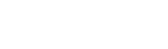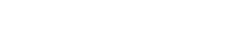# Category: Solutions## Integral of 1/sqrt(e^x-1) from ln(2) to infinity

Hi guys! Hope you are all doing well in these days of quarantine which is hopefully ending soon. I like to spend my time integrating and watching videos about calculus… Read more Integral of 1/sqrt(e^x-1) from ln(2) to infinity## Definite double integral of sin(y²)

I’ll be honest: I had no idea how to do this at first, but then I thought that it was probably easier than I could imagine. What most likely blocks… Read more Definite double integral of sin(y²)## Hard looking yet beautiful integral

Never judge a book by its cover: this is the case. This integral requires a bit of work and knowledge, as you need to know about the Gaussian integral (I… Read more Hard looking yet beautiful integral## Gaussian integral using Feynman’s technique

In my last post we evaluated the following definite integral This is the formula we got: and this is the integral we want to evaluate: which is equivalent to because… Read more Gaussian integral using Feynman’s technique## Integral of sin(x)/x from 0 to infinity | A satisfying solution

Today we have a tough integral: not only is this a special integral (the sine integral Si(x)), but it also goes from 0 to infinity! Because of the first characteristic,… Read more Integral of sin(x)/x from 0 to infinity | A satisfying solution## Cauchy principal value | Definite integral of 1/x

We know that the integral of 1/x is the natural logarithm ln(x). Very straightforward, yes, but it’s not as simple when it comes to evaluating the definite integral. As you… Read more Cauchy principal value | Definite integral of 1/x

{{#pages}} {{/pages}}
%%footer%%